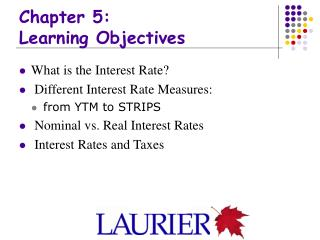DownloadDownload PresentationChapter 5: Learning Objectives

# Chapter 5: Learning Objectives

Télécharger la présentation## Chapter 5: Learning Objectives

- - - - - - - - - - - - - - - - - - - - - - - - - - - E N D - - - - - - - - - - - - - - - - - - - - - - - - - - -
##### Presentation Transcript

1. Chapter 5:Learning Objectives • What is the Interest Rate? • Different Interest Rate Measures: • from YTM to STRIPS • Nominal vs. Real Interest Rates • Interest Rates and Taxes

2. Payment Schemes • Simple loan: one payment at maturity • Fixed payment loan: payments at fixed intervals • Coupon bond: fixed payouts & principal repayment • Discounted bonds: Treasury bill • STRIPS: Separate Trading of Registered Principal & Interest of Securities

3. The Basic Present Value Principle \$X \$100 t t+1 Present Value Future Value

4. 2006 Present Value of \$100

5. The Coupon Bond PV = [\$C/(1+R)] + …+ [\$C/(1+R)n] + [\$FV/(1+R)n] \$C \$FV \$C \$C R R R t+n t+n-1 t t+1

6. Bond Prices and Interest Rates

7. Discounted Bills: The Treasury Bill R = [(\$FV - \$PD)/\$PD]X (360/90) Maturity period \$PD \$FV

8. Current Yield R = \$C/Pt + Pt/Pt Current Yield = one-period return + Capital gain/loss \$C Pt Pt+1

9. STRIPS Investors wanting a lump-sum payment will prefer option I I \$FV Pt Pt (1 + R)n = FV \$C \$C \$C \$C II \$C=% FV Investors wanting regular payments will want Option II

10. Internal Rate of Return \$NF0={\$NF1/(1+R)}+ {\$NF1/(1+R)2}+…+{\$NFn/(1+R)n} NF1 Initial Outlay of \$NF0 t=0 NFn Cash flows over n periods

11. Nominal vs. Real Interest Rates e e e Ex ante    t+1 t+3 t+2 Ex post R R=+e R=+ Nominal Interest rate fixed

12. Nominal Interest Rate and Inflation

13. Real Interest Rates in Canada

14. Financial Focus 5.2: Real Return Bonds

15. How the Real return bond works: An Example • Reference vs Actual Date • Need to interpolate because CPI is available monthly with a lag • Purchase date: 20 JAN 2003; Selling date: 20 July 2003 (= 6 month holding period) • Reference date is: 20 April 2003 • CPI 20 July 2003= (130.1 [CPI 30/4/03] * (20/31=interpolation period) + 129.9 [CPI 31/3/03])= 130.03 • Inflation during holding period is: (130.03-126.64)/126.64=2.68%

16. How the Real return bond works: An Example • Amounts received for a \$100,000 real return bond held for 6 months: • compensation for inflation: \$100,000*1.0268= \$102,677.94 • Compensation for real return: \$102,677.94*.02125 (1/2 of 4.25% real return) = \$2181.91 • TOTAL: \$102,677.94+ \$2181.91 = \$104,859.83

17. Summary • Debt is like a futures contract • The “price” of debt is the “interest rate” • The PV of a stream of payments is discounted by the interest rate until the term to maturity • The key mathematical relations are: • Xt = X0 / (1+R)n • PVt = [\$C/(1+R)] + … + [\$C/(1+R)n)] + [\$FV/(1+R)n] • R = (\$FV - \$PD)/ \$PD X (360/90) • Rct = C/ Pt • Fisher equation: R =  + 